Test: Data Interpretation- 2

# Test: Data Interpretation- 2

Test Description

## 20 Questions MCQ Test Quantitative Techniques for CLAT | Test: Data Interpretation- 2

Test: Data Interpretation- 2 for Quant 2022 is part of Quantitative Techniques for CLAT preparation. The Test: Data Interpretation- 2 questions and answers have been prepared according to the Quant exam syllabus.The Test: Data Interpretation- 2 MCQs are made for Quant 2022 Exam. Find important definitions, questions, notes, meanings, examples, exercises, MCQs and online tests for Test: Data Interpretation- 2 below.
Solutions of Test: Data Interpretation- 2 questions in English are available as part of our Quantitative Techniques for CLAT for Quant & Test: Data Interpretation- 2 solutions in Hindi for Quantitative Techniques for CLAT course. Download more important topics, notes, lectures and mock test series for Quant Exam by signing up for free. Attempt Test: Data Interpretation- 2 | 20 questions in 30 minutes | Mock test for Quant preparation | Free important questions MCQ to study Quantitative Techniques for CLAT for Quant Exam | Download free PDF with solutions
 1 Crore+ students have signed up on EduRev. Have you?
Test: Data Interpretation- 2 - Question 1

### The following pie-charts show the distribution of students of graduate and post-graduate levels in seven different institutes in a town. Distribution of students at graduate and post-graduate levels in seven institutes: Total number of students of graduate level = 27300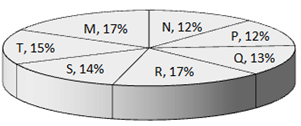Total number of students of post – graduate level = 24700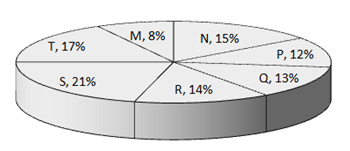Q. What is the total number of graduate and post-graduate level students in institute R?

Test: Data Interpretation- 2 - Question 2

### The following pie-charts show the distribution of students of graduate and post-graduate levels in seven different institutes in a town. Distribution of students at graduate and post-graduate levels in seven institutes: Total number of students of graduate level = 27300Total number of students of post – graduate level = 24700Q. What is the ratio between the number of students studying at post-graduate and graduate levels respectively from institute S?

Test: Data Interpretation- 2 - Question 3

### The following pie-charts show the distribution of students of graduate and post-graduate levels in seven different institutes in a town. Distribution of students at graduate and post-graduate levels in seven institutes: Total number of students of graduate level = 27300Total number of students of post – graduate level = 24700Q. How many students of institutes of M and S are studying at graduate level?

Test: Data Interpretation- 2 - Question 4

The following pie-charts show the distribution of students of graduate and post-graduate levels in seven different institutes in a town.
Distribution of students at graduate and post-graduate levels in seven institutes:
Total number of students of graduate level = 27300Total number of students of post – graduate level = 24700Q.

What is the ratio between the number of students studying at post-graduate level from institutes S and the number of students   studying at graduate level from institute Q?

Detailed Solution for Test: Data Interpretation- 2 - Question 4

The correct answer is D
as THE RATIO WIL BE
21% of 24700) / (13% of 27300)
=(21*247)/(13*273)
=(3*247)/(13*39)
=(3*19)/(1*39)
=19/13
Ratio = 19:13

Test: Data Interpretation- 2 - Question 5

The following pie-charts show the distribution of students of graduate and post-graduate levels in seven different institutes in a town.
Distribution of students at graduate and post-graduate levels in seven institutes:
Total number of students of graduate level = 27300Total number of students of post – graduate level = 24700Q.

Total number of students studying at post-graduate level from institutes N and P is

Test: Data Interpretation- 2 - Question 6

Study the following line graph and answer the questions.

Exports from Three Companies Over the Years (in Rs. crore)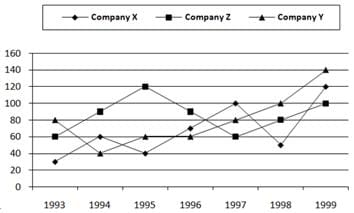Q.

For which of the following pairs of years the total exports from the three Companies together are equal?

Test: Data Interpretation- 2 - Question 7

Study the following line graph and answer the questions.

Exports from Three Companies Over the Years (in Rs. crore)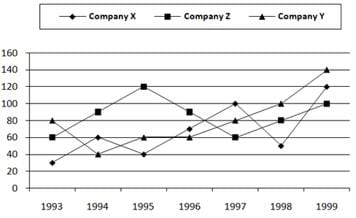Q.
Average annual exports during the given period for Company Y is approximately what percent of the average annual exports for Company Z?

Test: Data Interpretation- 2 - Question 8

Study the following line graph and answer the questions.

Exports from Three Companies Over the Years (in Rs. crore)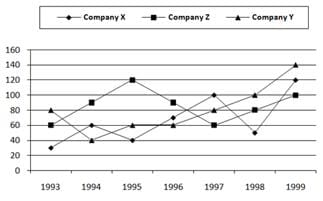Q.
In which year was the difference between the exports from Companies X and Y the minimum?

Test: Data Interpretation- 2 - Question 9

Study the following line graph and answer the questions.

Exports from Three Companies Over the Years (in Rs. crore)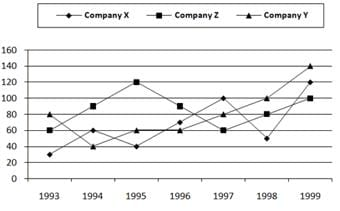Q.
What was the difference between the average exports of the three Companies in 1993 and the average exports in 1998?

Test: Data Interpretation- 2 - Question 10

Study the following line graph and answer the questions.

Exports from Three Companies Over the Years (in Rs. crore)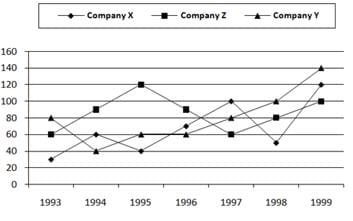Q.
In how many of the given years, were the exports from Company Z more than the average annual exports over the given   years?

Detailed Solution for Test: Data Interpretation- 2 - Question 10

Average annual exports of Company Z during the given period
= 1/7 x (60 + 90 + 120 + 90 + 60 + 80 + 100)
= Rs. ( 600 /7)crores
= Rs. 85.71 crores.
From the analysis of graph the exports of Company Z are more than the average annual exports of Company Z (i.e., Rs. 85.71 crores) during the years 1994, 1995, 1996 and 1999, i.e., during 4 of the given years.

Test: Data Interpretation- 2 - Question 11

Study the following line graph and answer the questions based on it.

Number of Vehicles Manufactured by Two companies over the Years (Number in Thousands)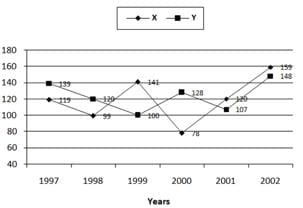Q.
What is the difference between the number of vehicles manufactured by Company Y in 2000 and 2001?

Detailed Solution for Test: Data Interpretation- 2 - Question 11

The correct is D as
in 2000 , Y had 128000 units
and in 2001 , Y had 107000 units
128000 - 107000 = 21000  , so correct option is D.

Test: Data Interpretation- 2 - Question 12

Study the following line graph and answer the questions based on it.

Number of Vehicles Manufactured by Two companies over the Years (Number in Thousands)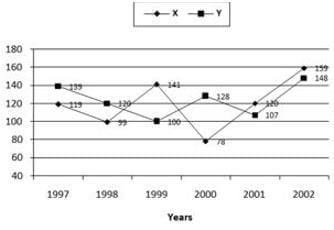Q.
What is the difference between the total productions of the two Companies in the given years?

Test: Data Interpretation- 2 - Question 13

Study the following line graph and answer the questions based on it.

Number of Vehicles Manufactured by Two companies over the Years (Number in Thousands)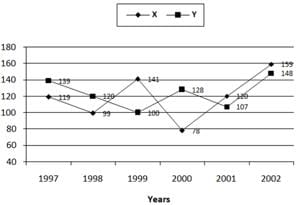Q.
What is the average numbers of vehicles manufactured by Company X over the given period? (rounded off to nearest integer)

Detailed Solution for Test: Data Interpretation- 2 - Question 13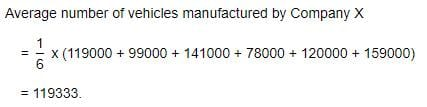Test: Data Interpretation- 2 - Question 14

Study the following line graph and answer the questions based on it.

Number of Vehicles Manufactured by Two companies over the Years (Number in Thousands)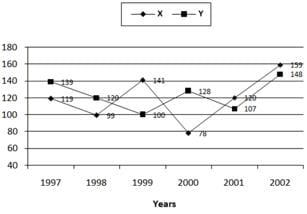Q.
In which of the following years, the difference between the productions of Companies X and Y was the maximum among the   given years?

Test: Data Interpretation- 2 - Question 15

Study the following line graph and answer the questions based on it.

Number of Vehicles Manufactured by Two companies over the Years (Number in Thousands)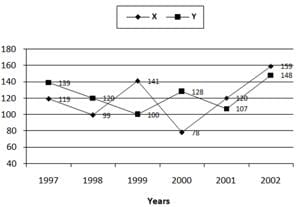Q.
The production of Company Y in 2000 was approximately what percent of the production of Company X in the same year?

Test: Data Interpretation- 2 - Question 16

The following line graph gives the percent profit earned by two Companies X and Y during the period 1996 - 2001.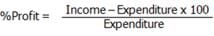Percentage profit earned by Two Companies X and Y over the Given Years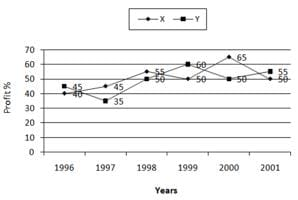Q.
The incomes of two Companies X and Y in 2000 were in the ratio of 3:4 respectively. What was the respective ratio of their   expenditures in 2000?

Detailed Solution for Test: Data Interpretation- 2 - Question 16

The correct answer is C
Let the incomes in 2000 of Companies X and Y be 3x and 4x respectively.
And let the expenditures in 2000 of Companies X and Y be E1 and E2 respectively.
Then, for Company X we have:
65 =[( 3x - E1 )/E1]×100 =>65/100 = (3x/E1) - 1
=> E1 = 3x×( 100/165 ) .... (i)
For Company Y we have:
50 = [(4x - E2 )/E2]x 100 =>50/100= (4x/E1) - 1 => E2 = 4x×( 100/150 ) .... (ii)
From (i) and (ii), we get:
E1/E2=[3x ×(100/165)]/[4x×(100/150)]
E1/E2=15/22 (required ratio)

Test: Data Interpretation- 2 - Question 17

The following line graph gives the percent profit earned by two Companies X and Y during the period 1996 - 2001.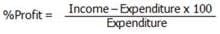Percentage profit earned by Two Companies X and Y over the Given Years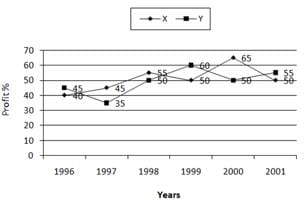Q.
If the expenditure of Company Y in 1997 was Rs. 220 crores, what was its income in 1997?

Detailed Solution for Test: Data Interpretation- 2 - Question 17

The correct anwer is B as profit percent of Company Y in 1997 = 35.
Let the income of Company Y in 1997 be Rs. x crores.
Then, 35 =
x - 220
x 100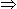x = 297.
220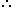Income of Company Y in 1997
= Rs. 297 crores.

Test: Data Interpretation- 2 - Question 18

The following line graph gives the percent profit earned by two Companies X and Y during the period 1996 - 2001.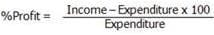Percentage profit earned by Two Companies X and Y over the Given Years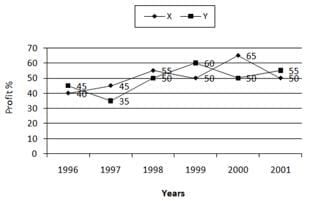Q.
If the expenditures of Company X and Y in 1996 were equal and the total income of the two Companies in 1996 was Rs. 342   crores, what was the total profit of the two Companies together in 1996? (Profit = Income - Expenditure)

Detailed Solution for Test: Data Interpretation- 2 - Question 18

The correct answer is D as ,
Total Income = 342 cr
Income in percentage = 140+145=285%
Expenditure per year= 200×342/285 = 240 cr
So total profit in 1996 = 342 - 240 = 102 cr.

Test: Data Interpretation- 2 - Question 19

The following line graph gives the percent profit earned by two Companies X and Y during the period 1996 - 2001.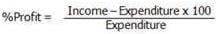Percentage profit earned by Two Companies X and Y over the Given Years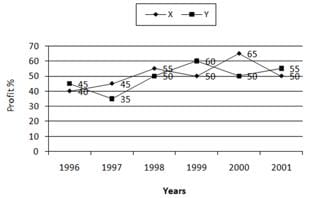Q.
The expenditure of Company X in the year 1998 was Rs. 200 crores and the income of company X in 1998 was the same as its expenditure in 2001. The income of Company X in 2001 was ?

Detailed Solution for Test: Data Interpretation- 2 - Question 19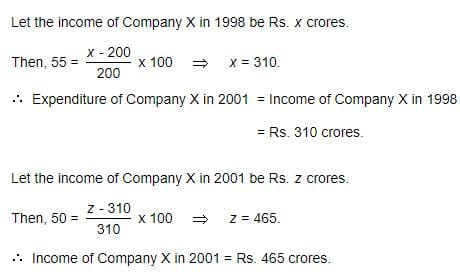Test: Data Interpretation- 2 - Question 20

The following line graph gives the percent profit earned by two Companies X and Y during the period 1996 - 2001.Percentage profit earned by Two Companies X and Y over the Given Years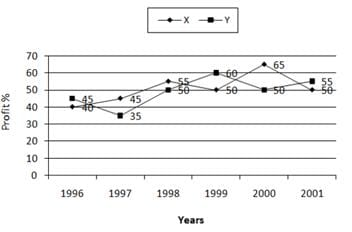Q.
If the incomes of two Companies were equal in 1999, then what was the ratio of expenditure of Company X to that of Company Y in 1999 ?

Detailed Solution for Test: Data Interpretation- 2 - Question 20

The correct answer is D
In 1999 , profit % of X = 50
profit% of Y = 60
Also , let their income in 1999 be i each and expenditure of X be x and that of Y be y.
For X ,
50 = (i - x)*100/x
1/2 = (i - x)/x
x/2 = i - x
i = 3x/2 (eq1)
For Y ,
60 = (i - y)*100/y
3/5 = (i - y)/y
3y/5 = i - y
i = 8y/5(eq 2)
Equating eq 1and 2 , we get
3x/2 = 8y/5
x/y = 16/15
x : y = 16:15

## Quantitative Techniques for CLAT

56 videos|35 docs|91 tests
 Use Code STAYHOME200 and get INR 200 additional OFF Use Coupon Code
Information about Test: Data Interpretation- 2 Page
In this test you can find the Exam questions for Test: Data Interpretation- 2 solved & explained in the simplest way possible. Besides giving Questions and answers for Test: Data Interpretation- 2 , EduRev gives you an ample number of Online tests for practice

## Quantitative Techniques for CLAT

56 videos|35 docs|91 tests